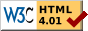#Boost C++ Libraries

...one of the most highly regarded and expertly designed C++ library projects in the world.

This is the documentation for an old version of boost. Click here for the latest Boost documentation.# for - m4 FOR Macro

## Introduction

detail/for.m4 provides BOOST_M4_FOR, an m4 macro that provides the equivalent of a #for preprocessor instruction.

## Usage

This macro must be used by an m4 file. This file assumes that the option -P is used, to force an m4_ prefix on all builtin symbols.

## Arguments

1. The name of a variable to hold the current value.
2. The starting value of the variable.
3. The ending value of the variable.
4. The text to repeat. This text may contain references to the variable, which will be replaced with the variable's current value.
5. The delimeter text (optional).

If called with the wrong number of arguments (less than 4 or more than 5), BOOST_M4_FOR will exit with an error. If the starting value (\$2) is greater than or equal to the ending value (\$3), BOOST_M4_FOR will do nothing. Otherwise, it will repeat the text (\$4), binding the variable (\$1) to the values in the range [starting value (\$2), ending value (\$3)), and repeat the delimeter text (\$5) in-between each occurrence of the repeat text (\$4).

## Examples

Note of the quotation marks (") used in the table below are in the input or output; they are shown to delimit whitespace. All code within a pair of quotation marks is intended to be on one line.

Input Output
"BOOST_M4_FOR(i, 1, 3)" Boost m4 script: BOOST_M4_FOR: Wrong number of arguments (3)
"BOOST_M4_FOR(i, 1, 3, i, ` ', 13)" Boost m4 script: BOOST_M4_FOR: Wrong number of arguments (6)
"BOOST_M4_FOR(i, 7, 0, i )" (nothing)
"BOOST_M4_FOR(i, 0, 0, i )" (nothing)
"BOOST_M4_FOR(i, 0, 7, i )" "0 1 2 3 4 5 6 "
"BOOST_M4_FOR(i, -13, -10, i )" "-13 -12 -11 "
"BOOST_M4_FOR(i, 0, 8, BOOST_M4_FOR(j, 0, 4, (i, j) )"
")"
"(0, 0) (0, 1) (0, 2) (0, 3) "
"(1, 0) (1, 1) (1, 2) (1, 3) "
"(2, 0) (2, 1) (2, 2) (2, 3) "
"(3, 0) (3, 1) (3, 2) (3, 3) "
"(4, 0) (4, 1) (4, 2) (4, 3) "
"(5, 0) (5, 1) (5, 2) (5, 3) "
"(6, 0) (6, 1) (6, 2) (6, 3) "
"(7, 0) (7, 1) (7, 2) (7, 3) "
""
"BOOST_M4_FOR(i, 7, 0, i, |)" (nothing)
"BOOST_M4_FOR(i, 0, 0, i, |)" (nothing)
"BOOST_M4_FOR(i, 0, 7, i, |)" "0|1|2|3|4|5|6"
"BOOST_M4_FOR(i, -13, -10, i, `, ')" "-13, -12, -11"
"BOOST_M4_FOR(i, 0, 8, `[BOOST_M4_FOR(j, 0, 4, (i, j), `, ')]', `,"
"')"
"[(0, 0), (0, 1), (0, 2), (0, 3)],"
"[(1, 0), (1, 1), (1, 2), (1, 3)],"
"[(2, 0), (2, 1), (2, 2), (2, 3)],"
"[(3, 0), (3, 1), (3, 2), (3, 3)],"
"[(4, 0), (4, 1), (4, 2), (4, 3)],"
"[(5, 0), (5, 1), (5, 2), (5, 3)],"
"[(6, 0), (6, 1), (6, 2), (6, 3)],"
"[(7, 0), (7, 1), (7, 2), (7, 3)]"Revised 05 December, 2006